# KSEEB Solutions for Class 5 Maths Chapter 5 Fractions

Students can Download Maths Chapter 5 Fractions Questions and Answers, Summary, Notes Pdf, KSEEB Solutions for Class 5 Maths helps you to revise the complete Karnataka State Board Syllabus and score more marks in your examinations.

## Karnataka State Syllabus Class 5 Maths Chapter 5 Fractions

### KSEEB Class 5 Maths Fractions Ex 5.1

Question 1.
Which of the following figures have been divided into parts of the same size?b & c.Question 2.
Draw a line to divide each of these figures into the required number of equal parts.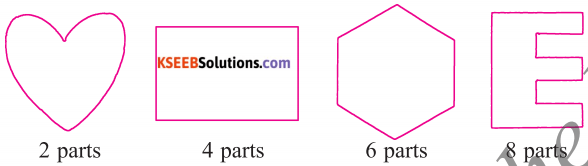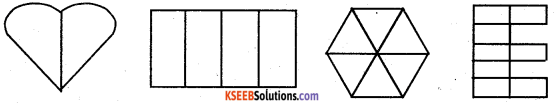This online Least Common Denominator Calculator (or LCD calculator) calculates the least common denominator (or the lowest common denominator) of fractions.

Question 3.
Write each of the following as a fraction
a) Half –$$\frac{1}{2}$$
b) Two-third – $$\frac{2}{3}$$
c) Two-tenth – $$\frac{2}{10}$$
d) Five-seventh – $$\frac{5}{7}$$
e) Five-sixteenth – $$\frac{5}{16}$$
f) Five-twelveth – $$\frac{5}{12}$$
g) Eight-nineth – $$\frac{8}{9}$$
h) Four-nineth – $$\frac{4}{9}$$
i) Three-fourth – $$\frac{3}{4}$$
j) Two-fifth – $$\frac{2}{5}$$Question 4.
Write each of the fraction in words
a) $$\frac{2}{5}$$ – two-fifth
b) $$\frac{3}{4}$$ – three-fourth
c) $$\frac{7}{10}$$ – seven-tenth
d) $$\frac{11}{12}$$ – eleven-twelveth
e) $$\frac{2}{3}$$ – two-third
f) $$\frac{4}{5}$$ – four-fifth
g) $$\frac{5}{8}$$ – five-eight
h) $$\frac{3}{7}$$ – three-seventh
i) $$\frac{5}{6}$$ – five-sixth
j) $$\frac{7}{9}$$ – seven-ninethQuestion 5.
What fraction of the figure is shaded?
a) $$\frac{2}{3}$$b) $$\frac{2}{4}$$c) $$\frac{5}{8}$$d) $$\frac{7}{12}$$e) $$\frac{7}{15}$$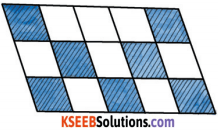f) $$\frac{6}{18}$$Question 6.
Fill in the blanks:
a) The denominator of the fraction $$\frac{1}{8}$$ is 8
b) The numerator of the fraction is $$\frac{2}{5}$$ 2
c) 3 is the denominator of fraction $$\frac{1}{3}$$
d) 1 is the numerater of the fraction $$\frac{1}{5}$$
e) In a fraction, the denominator is written below the numerator separated by a line.Question 7.
Write down the fraction of the coloured portions in these figures.
a)$$\frac{1}{5}$$

b)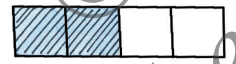$$\frac{2}{4}$$

c)$$\frac{4}{12}$$

d)$$\frac{3}{6}$$

e)$$\frac{4}{9}$$Question 8.
Write the fraction for the shaded part in each of the following figures.$$\frac{4}{7}$$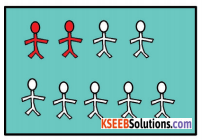$$\frac{2}{9}$$$$\frac{7}{10}$$Question 9.
Colour the figures as instructed.

Eg: $$\frac{7}{10}$$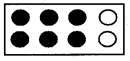$$\frac{1}{5}$$$$\frac{2}{3}$$$$\frac{2}{9}$$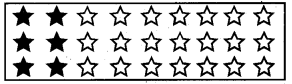Question 10.
Represent the given fraction by drawing a line.
a) What is $$\frac{1}{3}$$ of 12?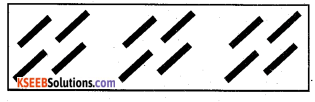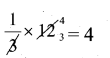b) What is $$\frac{2}{5}$$ of 15?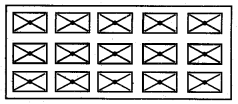c) What is $$\frac{2}{7}$$ of 21?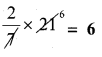Question 11.
Shade the figures to show the fractions.
a) $$\frac{6}{11}$$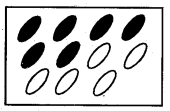b) $$\frac{7}{20}$$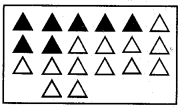c) $$\frac{8}{15}$$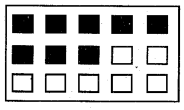Question 12.
Which of the following shaded figures represent $$\frac{4}{9}$$ ?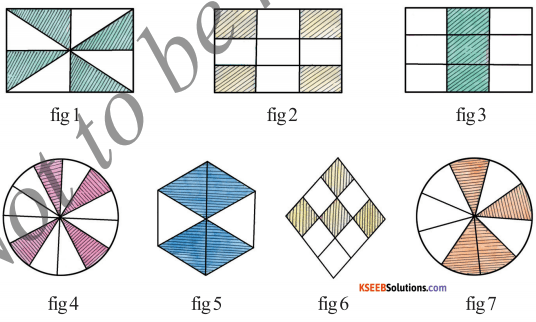Question 13.
Which of the following shaded figure represents $$\frac{5}{12}$$ and give reason.fig b&d, Reason: 5 objectives out of 12 are shaded

Question 14.
Represent the grouped parts as fractions:
a)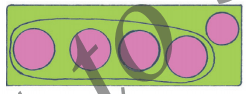$$\frac{4}{5}$$

b)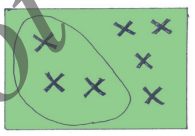$$\frac{3}{7}$$

c)$$\frac{5}{6}$$### KSEEB Class 5 Maths Fractions Ex 5.2

I. Fill in the gaps using > or < signs.### KSEEB Class 5 Maths Fractions Ex 5.3

I. Complete the series.2 × 2 = 4
2 × 3 = 6

3 × 2 =6
3 × 3 = 9
3 × 4 = 12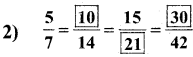5 × 2 = 10
7 × 2 = 14

5 × 3 = 15
7 × 3 = 21

5 × 6 = 30
7 × 6 = 42II. Write the next three equivalent fractions.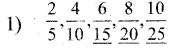2 × 3 = 6, 5 × 3 = 15
2 × 4 = 8, 5 × 4 = 20
2 × 5 = 10, 5 × 5 = 253 × 3 = 9
3 × 4 = 12
3 × 5 = 15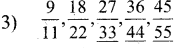9 × 3 = 27, 11 × 3 = 33
9 × 4 = 36, 11 × 4 = 44
9 × 5 = 45, 11 × 5 = 55III. Are the two fractions equivalent?

Question 1.
$$\frac{3}{5}$$ and $$\frac{18}{30}$$
Yes,Reason, $$\frac{3}{5} \times \frac{6}{6}=\frac{18}{30}$$

Question 2.
$$\frac{12}{17}$$ and $$\frac{8}{20}$$
No, Both terms are different

Question 3.
$$\frac{2}{7}$$ and $$\frac{7}{21}$$”
No, Both terms are different

Question 4.
$$\frac{5}{11}$$ and $$\frac{25}{55}$$
Yes,Reason, $$\frac{5 \times 5}{11 \times 5}=\frac{25}{55}$$IV. Find an equivalent raction of $$\frac{4}{7}$$ having:

1. 16 as numerator
$$\frac{4 \times 4}{7 \times 4}=\frac{16}{28}$$

2. 24 as numerator
$$\frac{4 \times 6}{7 \times 6}=\frac{24}{42}$$

3. 21 as denominator
$$\frac{4 \times 3}{7 \times 3}=\frac{12}{24}$$

4. 84 as denominator
$$\frac{4 \times 12}{7 \times 12}=\frac{48}{84}$$### KSEEB Class 5 Maths Fractions Ex 5.5

I. Reduce each of the following fractions into its lowest form.

1. $$\frac{8}{16}=\frac{1}{2}$$
2. $$\frac{5}{10}=\frac{1}{2}$$
3. $$\frac{54}{108}=\frac{1}{2}$$
4. $$\frac{4}{20}=\frac{1}{5}$$
5. $$\frac{3}{15}=\frac{1}{5}$$
6. $$\frac{12}{16}=\frac{3}{4}$$
7. $$\frac{9}{27}=\frac{1}{3}$$
8. $$\frac{36}{48}=\frac{3}{4}$$
9. $$\frac{24}{56}=\frac{3}{7}$$
10. $$\frac{24}{72}=\frac{1}{3}$$### KSEEB Class 5 Maths Fractions Additional Questions

Question 1.
Estimate the degree of closeness of the fractions $$\frac{2}{7}, \frac{3}{7}$$ and $$\frac{5}{7}$$ and $$\frac{1}{4}, \frac{1}{2}$$ and $$\frac{3}{4}$$$$\frac{2}{7}$$ is closer to $$\frac{1}{4}, \frac{3}{7}$$ is closer to $$\frac{1}{2}$$

$$\frac{5}{7}$$ is closer to $$\frac{3}{4}$$

Question 2.
Estimate the degree of closeness of the fractions $$\frac{2}{9}, \frac{4}{9}, \frac{5}{9}$$ and $$\frac{7}{9}$$ to $$\frac{1}{4}, \frac{1}{2}$$ and $$\frac{3}{4}$$ .$$\frac{2}{9}$$ is closer to $$\frac{1}{4}, \frac{4}{9}$$ is closer to $$\frac{1}{2}$$
$$\frac{5}{9}$$ is closer to $$\frac{1}{2}, \frac{7}{9}$$ is closer to $$\frac{3}{4}$$